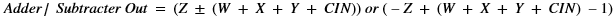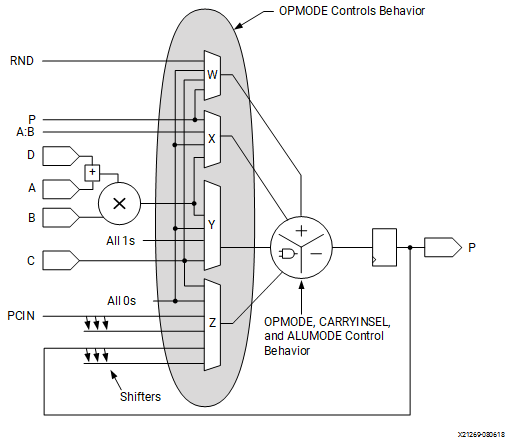# Simplified DSP58 Operations

## Versal ACAP DSP Engine Architecture Manual (AM004)

Document ID
AM004
Release Date
2022-09-11
Revision
1.2.1 English

The fixed-point math portion of DSP58 consists of a 27-bit pre-adder, a 27-bit by 24-bit two’s complement multiplier with optional product negation followed by four 58-bit datapath multiplexers (with outputs W, X, Y, and Z). This is followed by a four-input adder/subtracter or two-input logic unit. When using two-input logic unit, the multiplier cannot be used.

The data and control inputs to DSP58 feed the arithmetic and logic stages. The A and B data inputs can optionally be registered one or two times to assist the construction of different, highly pipelined, DSP application solutions. The D path and the AD path can each be registered once. The other data inputs and the control inputs can be optionally registered once.

The following equation summarizes the combination of W, X, Y, Z, and CIN by the adder/subtracter. The CIN, W multiplexer output, X multiplexer output, and Y multiplexer output are always added together. This combined result can be selectively added to or subtracted from the Z multiplexer output. The second option is obtained by setting the ALUMODE to `0001`.A typical use of DSP58 is where A and B inputs are multiplied and the result is added to or subtracted from the C register. Selecting the multiplier function consumes both X and Y multiplexer outputs to feed the adder. The two 51-bit partial products from the multiplier are sign-extended to 58 bits before being sent to the adder/subtracter.

When not using the first stage multiplier, the 58-bit, dual input, bit-wise logic function implements AND, OR, NOT, NAND, NOR, XOR, and XNOR. The inputs to these functions are:

• All 0s on the W multiplexer
• Either A:B or P on the X multiplexer
• Either all 1s or all 0s on the Y multiplexer depending on logic operation
• Either C, P, or PCIN on the Z multiplexer

Creating wider logic operations is feasible using this cascade path because PCIN is a cascade input from a lower DSP58. A 58-bit, triple input, bit-wise XOR3 logic operation is supported when the Y multiplexer selects the C input and ALUMODE[3:0] = `0100`.

The output of the adder/subtracter or logic unit feeds the pattern detector logic. The pattern detector allows DSP58 to support convergent rounding, counter autoreset when a count value has been reached, and overflow, underflow, and saturation in accumulators. In conjunction with the logic unit, the pattern detector can be extended to perform a 58-bit dynamic comparison of two 58-bit fields.

The following figure illustrates DSP58 in a simplified form. The nine OPMODE bits control the selection of the W, X, Y, and Z multiplexers, feeding the inputs to the adder, subtracter, or logic unit. In all cases, the 51-bit partial product data from the multiplier to the X and Y multiplexers is sign-extended, forming 58-bit input datapaths to the adder/subtracter. Based on 51-bit operands and a 58-bit accumulator output, the number of guard bits (that is, bits available to guard against overflow) is 7. To extend the number of MACC operations, the MACC_EXTEND feature must be used. This feature allows the MACC to extend to 116 bits with two DSP58s. If both A and B are limited to 18 bits (sign-extended to 27 and 24), then there are 22 (58–36) guard bits for the MACC. The CARRYOUT bits are invalid during multiply operations. Combinations of OPMODE, ALUMODE, CARRYINSEL, and CARRYIN control the function of the adder/subtracter or logic unit.

Figure 1. Simplified DSP58 Operation